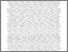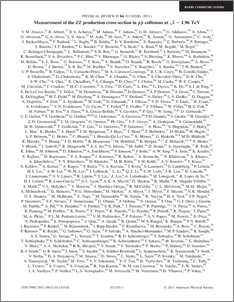# Measurement of the ZZ production cross section in p-pbar collisions at sqrt(s)=1.96 TeV

Collaboration, D0 and Bertram, Iain and Borissov, Guennadi and Fox, Harald and Ross, Anthony and Williams, Mark and Ratoff, Peter (2011) Measurement of the ZZ production cross section in p-pbar collisions at sqrt(s)=1.96 TeV. Physical Review D, 84 (1). ISSN 1550-7998Preview
PDF
PhysRevD.84.011103.pdf - Published Version

## Abstract

We present a new measurement of the production cross section $\sigma(p\bar{p} \rightarrow ZZ)$ at a center-of-mass energy $\sqrt{s}=1.96$ TeV, obtained from the analysis of the four charged lepton final state $\ell^{+}\ell^{-}\ell^{'+} \ell^{'-}$ ($\ell$, $\ell^{'}$ = $e$ or $\mu$). We observe ten candidate events with an expected background of $0.37 \pm 0.13$ events. The measured cross section $\sigma(p\bar{p} \to ZZ)= 1.26^{+0.47}_{-0.37} \mathrm{(stat)} \pm 0.14 \mathrm{(syst)}$ pb is in agreement with NLO QCD predictions. This result is combined with a previous result from the $ZZ\to\ell^{+}\ell^{-}\nu\bar{\nu}$ channel resulting in a combined cross section of $\sigma(p\bar{p} \to ZZ)= 1.40^{+0.43}_{-0.37} \mathrm{(stat)} \pm 0.14 \mathrm{(syst)}$ pb.

Item Type:
Journal Article
Journal or Publication Title:
Physical Review D